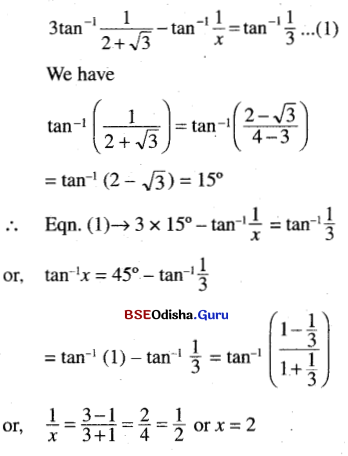# CHSE Odisha Class 12 Math Solutions Chapter 2 Inverse Trigonometric Functions Ex 2

Odisha State Board Elements of Mathematics Class 12 Solutions CHSE Odisha Chapter 2 Inverse Trigonometric Functions Ex 2 Textbook Exercise Questions and Answers.

## CHSE Odisha Class 12 Math Solutions Chapter 2 Inverse Trigonometric Functions Exercise 2

Question 1.
Fill in the blanks choosing correct answer from the brackets:
(i) If A = tan-1 x, then the value of sin 2A = ________. ($$\frac{2 x}{1-x^2}$$, $$\frac{2 x}{\sqrt{1-x^2}}$$, $$\frac{2 x}{1+x^2}$$)
Solution:
$$\frac{2 x}{1+x^2}$$

(ii) If the value of sin-1 x = $$\frac{\pi}{5}$$ for some x ∈ (-1, 1) then the value of cos-1 x is ________. ($$\frac{3 \pi}{10}$$, $$\frac{5 \pi}{10}$$,$$\frac{3 \pi}{10}$$)
Solution:
$$\frac{3 \pi}{10}$$

(iii) The value of tan-1 x (2cos$$\frac{\pi}{3}$$) is ________. (1, $$\frac{\pi}{4}$$, $$\frac{\pi}{3}$$)
Solution:
$$\frac{\pi}{4}$$

(iv) If x + y = 4, xy = 1, then tan-1 x + tan-1 y = ________. ($$\frac{3 \pi}{4}$$, $$\frac{\pi}{4}$$, $$\frac{\pi}{3}$$)
Solution:
$$\frac{\pi}{2}$$

(v) The value of cot-1 2 + tan-1 $$\frac{1}{3}$$ = ________. ($$\frac{\pi}{4}$$, 1, $$\frac{\pi}{2}$$)
Solution:
$$\frac{\pi}{4}$$

(vi) The principal value of sin-1 (sin $$\frac{2 \pi}{3}$$) is ________. ($$\frac{2 \pi}{3}$$, $$\frac{\pi}{3}$$, $$\frac{4 \pi}{3}$$)
Solution:
$$\frac{\pi}{3}$$(vii) If sin-1 $$\frac{x}{5}$$ + cosec-1 $$\frac{5}{4}$$ = $$\frac{\pi}{2}$$, then the value of x = ________. (2, 3, 4)
Solution:
x = 3

(viii) The value of sin (tan-1 x + tan-1 $$\frac{1}{x}$$), x > 0 = ________. (0, 1, 1/2)
Solution:
1

(ix) cot-1 $$\left[\frac{\sqrt{1-\sin x}+\sqrt{1+\sin x}}{\sqrt{1-\sin x}-\sqrt{1+\sin x}}\right]$$ = ________. (2π – $$\frac{x}{2}$$, $$\frac{x}{2}$$, π – $$\frac{x}{2}$$)
Solution:
π – $$\frac{x}{2}$$

(x) 2sin-1 $$\frac{4}{5}$$ + sin-1 $$\frac{24}{25}$$ = ________. (π, -π, 0)
Solution:
π

(xi) if Θ = cos-1 x + sin-1 x – tan-1 x, x ≥ 0, then the smallest interval in which Θ lies is ________. [($$\frac{\pi}{2}$$, $$\frac{3 \pi}{2}$$), [0, $$\frac{\pi}{2}$$), (0, $$\frac{\pi}{2}$$])
Solution:
(0, $$\frac{\pi}{2}$$]

(xii) sec2 (tan-1 2) + cosec2 (cot-1 3) = ________. (16, 14, 15)
Solution:
15

Question 2.
Write whether the following statements are true or false.
(i) sin-1 $$\frac{1}{x}$$ cosec-1 x = 1
Solution:
False

(ii) cos-1 $$\frac{4}{5}$$ + tan-1 $$\frac{2}{3}$$ = tan-1 $$\frac{17}{6}$$
Solution:
True

(iii) tan-1 $$\frac{4}{3}$$ + cot-1 ($$\frac{-3}{4}$$) = π
Solution:
True

(iv) sec-1 $$\frac{1}{2}$$ + cosec-1 $$\frac{1}{2}$$ = $$\frac{\pi}{2}$$
Solution:
False

(v) sec-1 (-$$\frac{7}{5}$$) = π – cos-1 $$\frac{5}{7}$$
Solution:
True

(vi) tan-1 (tan 3) = 3
Solution:
False

(vii) The principal value of tan-1 (tan $$\frac{3 \pi}{4}$$) is $$\frac{3 \pi}{4}$$
Solution:
False

(viii) cot-1 (-√3) is in the second quadrant.
Solution:
True

(ix) 3 tan-1 3 = tan-1 $$\frac{9}{13}$$
Solution:
False

(x) tan-1 2 + tan-1 3 = – $$\frac{\pi}{4}$$
Solution:
False

(xi) 2 sin-1 $$\frac{4}{5}$$ = sin-1 $$\frac{24}{25}$$
Solution:
False

(xii) The equation tan-1 (cotx) = 2x has exactly two real solutions.
Solution:
TrueQuestion 3.
Express the value of the foilowing in simplest form.
(i) sin (2 sin-1 0.6)
Solution:
sin (2 sin-1 0.6)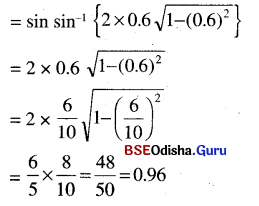(ii) tan ($$\frac{\pi}{4}$$ + 2 cot-1 3)
Solution: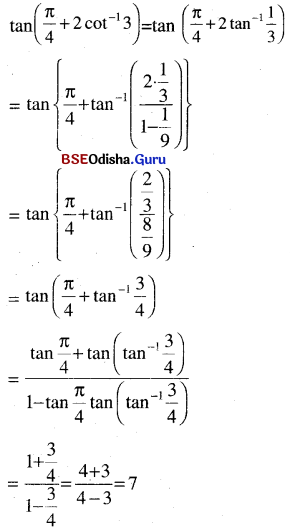(iii) cos (2 sin-1 x)
Solution: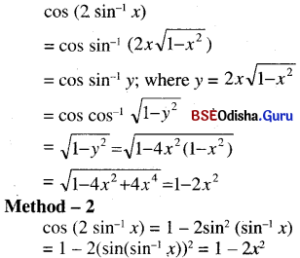(iv) tan (cos-1 x)
Solution: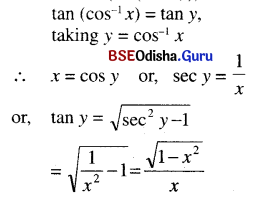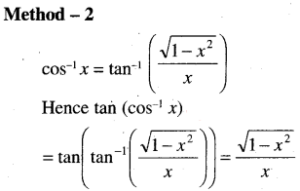(v) tan-1 ($$\frac{x}{y}$$) – tan-1 $$\frac{x-y}{x+y}$$
Solution: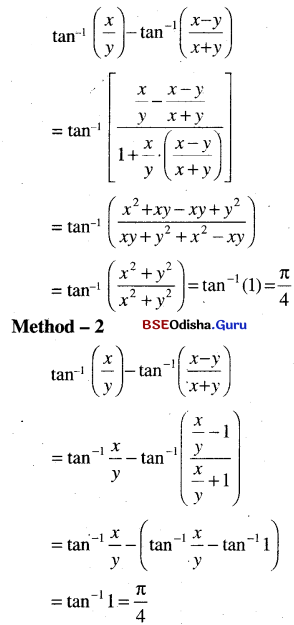(vi) cosec (cos-1 $$\frac{3}{5}$$ + cos-1 $$\frac{4}{5}$$)
Solution: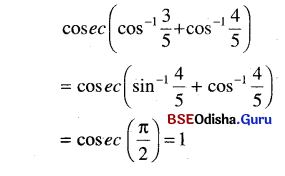(vii) sin-1 $$\frac{1}{\sqrt{5}}$$ + cos-1 $$\frac{3}{\sqrt{10}}$$
Solution: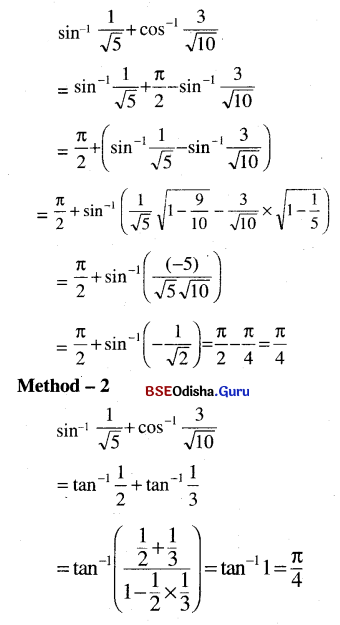(viii) sin cos-1 tan sec √2
Solution:
sin cos-1 tan sec √2
= sin cos-1 tan sec $$\frac{\pi}{4}$$
= sin cos-1 1 = sin 0 = 0

(ix) sin (2 tan-1 $$\sqrt{\frac{1-x}{1+x}}$$)
Solution: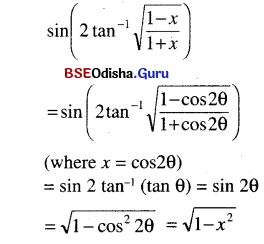(x) tan $$\left\{\frac{1}{2} \sin ^{-1} \frac{2 x}{1+x^2}+\frac{1}{2} \cos ^{-1} \frac{1-y^2}{1+y^2}\right\}$$
Solution: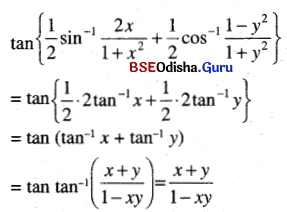(xi) sin cot-1 cos tan-1 x.
Solution: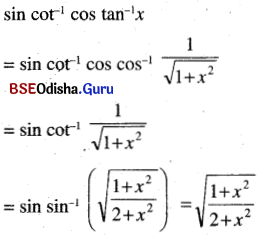(xii) tan-1 $$\left(x+\sqrt{1+x^2}\right)$$
Solution: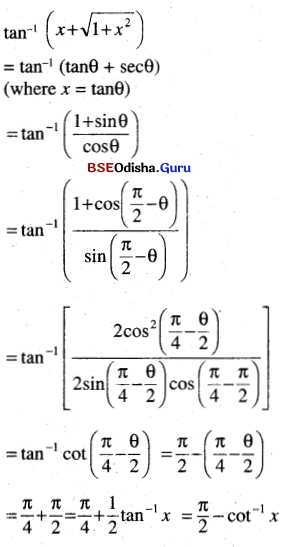Question 4.
Prove the following statements:
(i) sin-1 $$\frac{3}{5}$$ + sin-1 $$\frac{8}{17}$$ = cos-1 $$\frac{36}{85}$$
Solution: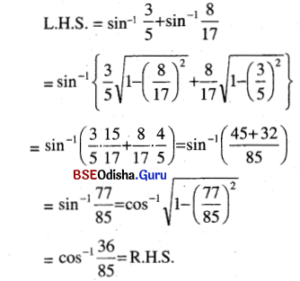(ii) sin-1 $$\frac{3}{5}$$ + cos-1 $$\frac{12}{13}$$ = cos-1 $$\frac{33}{65}$$
Solution: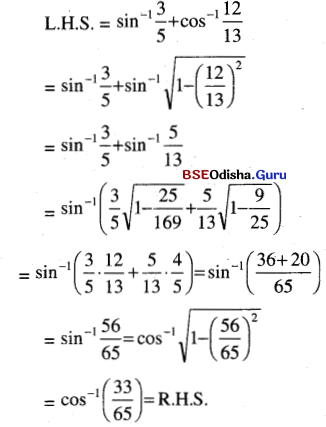(iii) tan-1 $$\frac{1}{7}$$ + tan-1 $$\frac{1}{13}$$ = tan-1 $$\frac{2}{9}$$
Solution:
L.H.S = tan-1 $$\frac{1}{7}$$ + tan-1 $$\frac{1}{13}$$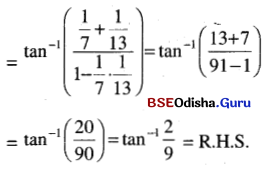(iv) tan-1 $$\frac{1}{2}$$ + tan-1 $$\frac{1}{5}$$ + tan-1 $$\frac{1}{8}$$ = $$\frac{\pi}{4}$$
Solution: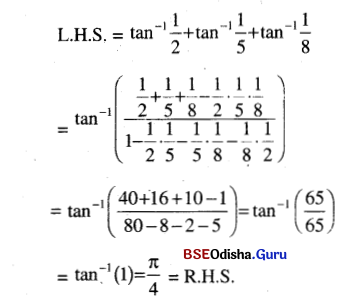(v) tan ( 2tan-1 $$\frac{1}{5}$$ – $$\frac{\pi}{4}$$ ) + $$\frac{7}{17}$$ = 0
Solution: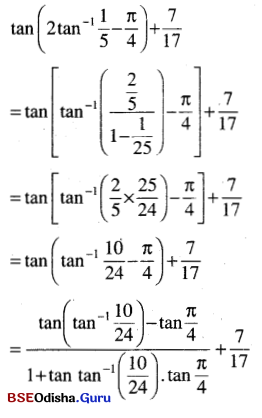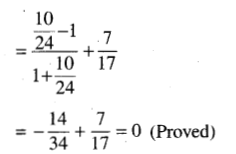Question 5.
Prove the following statements:
(i) cot-1 9 + cosec-1 $$\frac{\sqrt{41}}{4}$$ = $$\frac{\pi}{4}$$
Solution: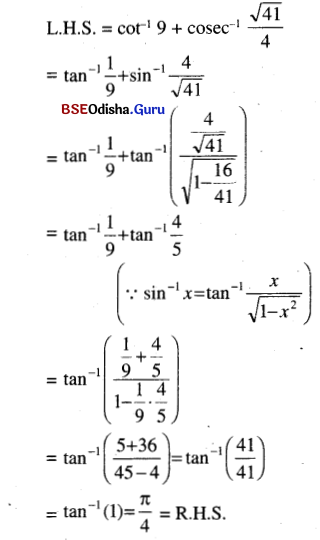(ii) sin-1 $$\frac{4}{5}$$ + 2 tan-1 $$\frac{1}{3}$$ = $$\frac{\pi}{2}$$
Solution: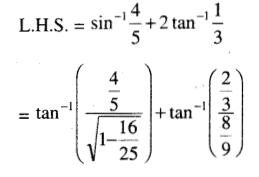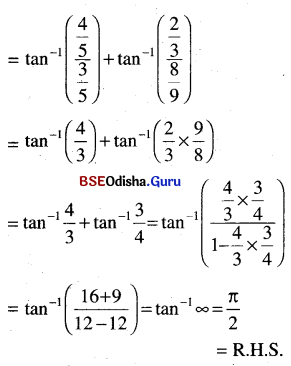(iii) 4 tan-1 $$\frac{1}{5}$$ – tan-1 $$\frac{1}{70}$$ + tan-1 $$\frac{1}{99}$$ = $$\frac{\pi}{4}$$
Solution: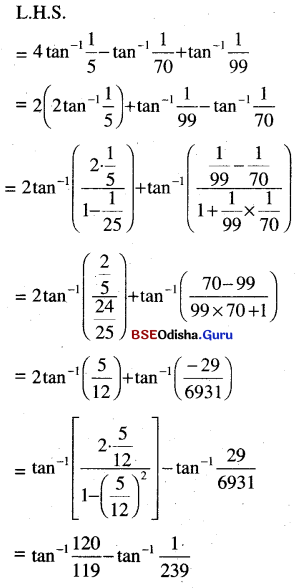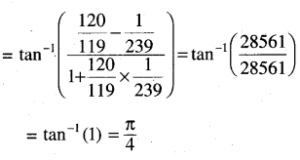(iv) 2 tan-1 $$\frac{1}{5}$$ + sec-1 $$\frac{5 \sqrt{2}}{7}$$ + 2 tan-1 $$\frac{1}{8}$$ = $$\frac{\pi}{4}$$
Solution: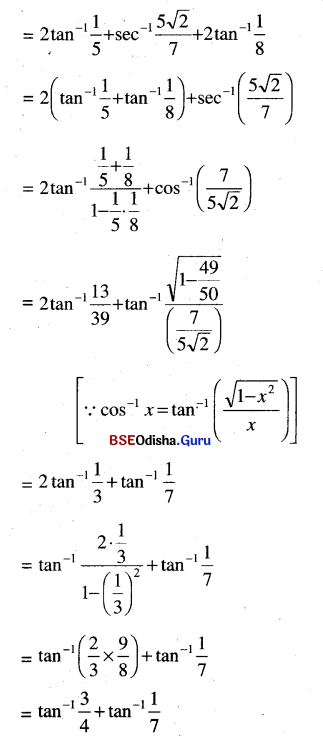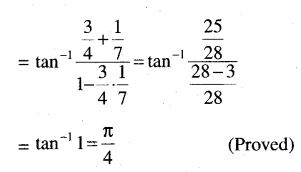(v) cos-1 $$\frac{12}{13}$$ + 2 cos-1 $$\sqrt{\frac{64}{65}}$$ + cos-1 $$\sqrt{\frac{49}{50}}$$ = cos-1 $$\frac{1}{\sqrt{2}}$$
Solution: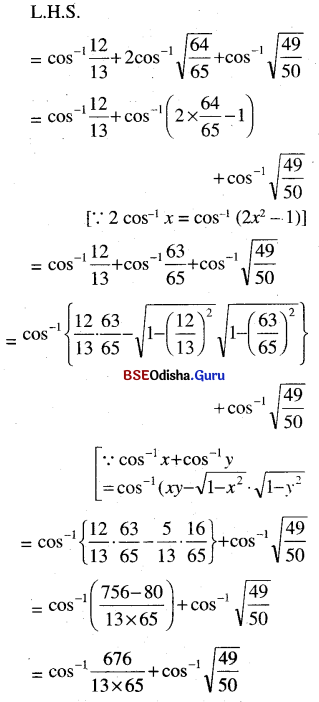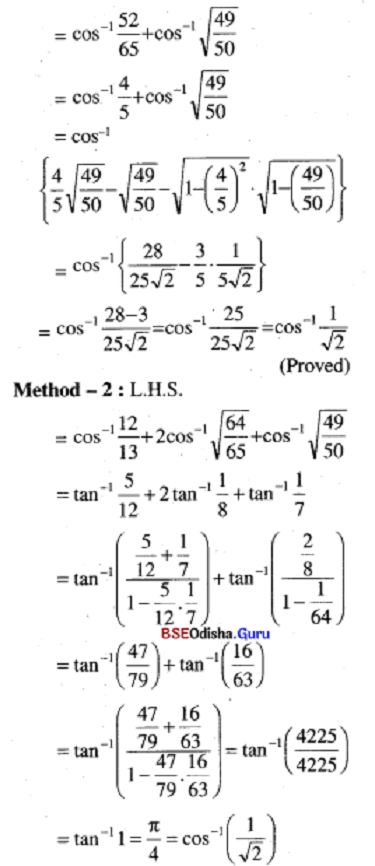(vi) tan2 cos-1 $$\frac{1}{\sqrt{3}}$$ + cot2 sin-1 $$\frac{1}{\sqrt{5}}$$ = 6
Solution:
tan2 cos-1 $$\frac{1}{\sqrt{3}}$$ + cot2 sin-1 $$\frac{1}{\sqrt{5}}$$
= tan2 tan-1 √2 + cot2 cot-1 (2)
= 2 + 4 = 6(vii) cos tan-1 cot sin-1 x = x.
Solution.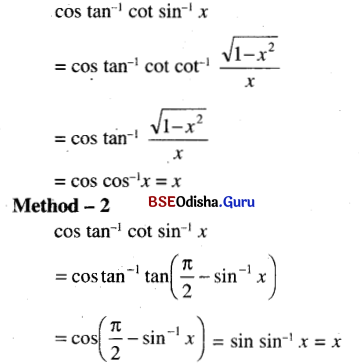Question 6.
Prove the following statements:
(i) cot-1 (tan 2x) + cot-1 (- tan 2x) = π
Solution: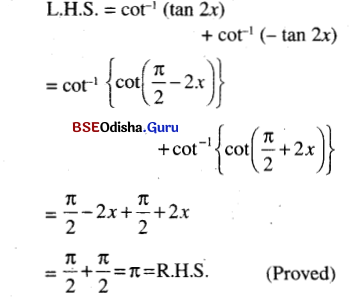(ii) tan-1 x + cot-1 (x + 1) = tan-1 (x2 + x + 1)
Solution: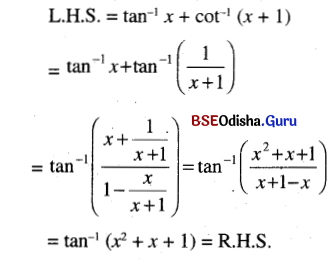(iii) tan-1 ($$\frac{a-b}{1+a b}$$) + tan-1 ($$\frac{b-c}{1+b c}$$) = tan-1 a – tan-1 c.
Solution:
tan-1 ($$\frac{a-b}{1+a b}$$) + tan-1 ($$\frac{b-c}{1+b c}$$)
= tan-1 a – tan-1 b + tan-1 b – tan-1 c
= tan-1 a – tan-1 c.

(iv) cot-1 $$\frac{p q+1}{p-q}$$ + cot-1 $$\frac{q r+1}{q-r}$$ + cot-1 $$\frac{r p+1}{r-p}$$ = 0
Solution: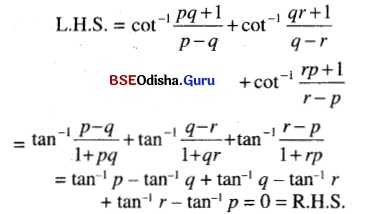(v)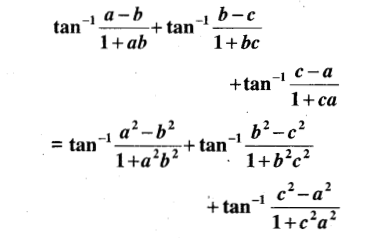Solution: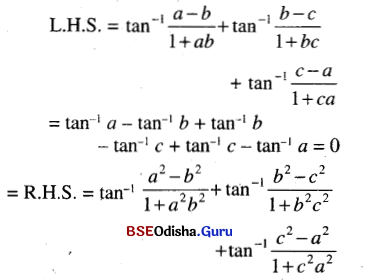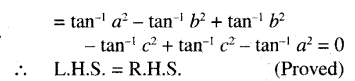Question 7.
Prove the following statements:
(i) tan-1 $$\frac{2 a-b}{b \sqrt{3}}$$ + tan-1 $$\frac{2 b-a}{a \sqrt{3}}$$ = $$\frac{\pi}{3}$$
Solution: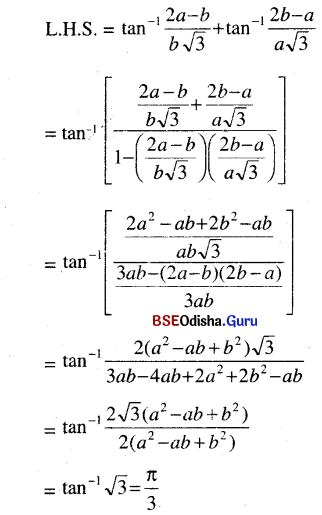(ii) tan-1 $$\frac{1}{x+y}$$ + tan-1 $$\frac{y}{x^2+x y+1}$$ = tan-1 $$\frac{1}{x}$$
Solution: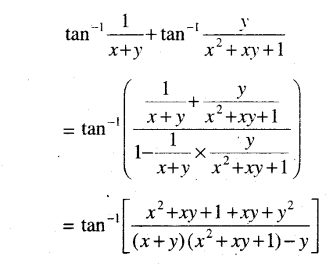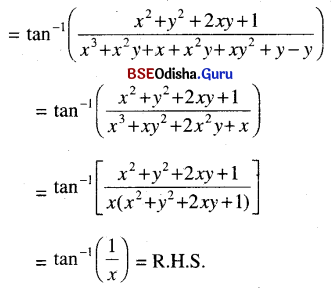(iii) sin-1 $$\sqrt{\frac{x-q}{p-q}}$$ = cos-1 $$\sqrt{\frac{p-x}{p-q}}$$ = cot-1 $$\sqrt{\frac{p-x}{x-q}}$$
Solution: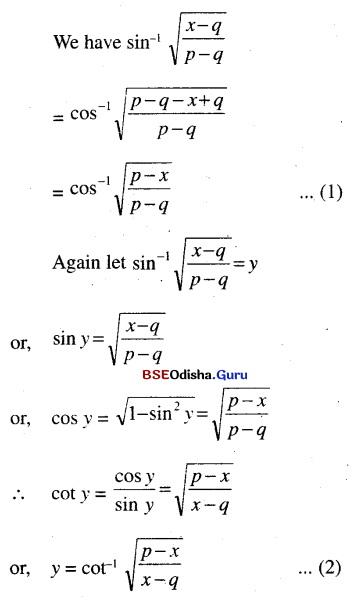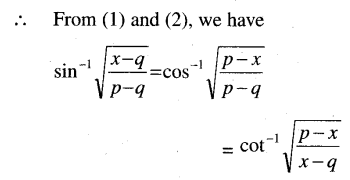(iv) sin2 (sin-1 x + sin-1 y + sin-1 z) = cos2 (cos-1 x + cos-1 y + cos-1 z)
Solution: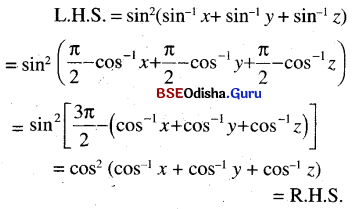(v) tan (tan-1 x + tan-1 y + tan-1 z) = cot (cot-1 x + cot-1 y + cot-1 z)
Solution: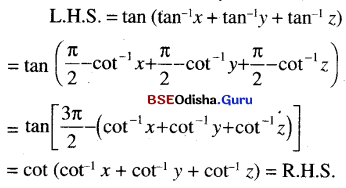Question 8.
(i) If sin-1 x + sin-1 y + sin-1 z = π, show that x$$\sqrt{1-x^2}$$ + x$$\sqrt{1-y^2}$$ + x$$\sqrt{1-z^2}$$ = 2xyz
Solution:
Let sin-1 x = α, sin-1 y = β, sin-1 z = γ
∴ α + β + γ = π
∴ x = sin α, y = sin β, z = sin γ
or, α + β = π – γ
or, sin(α + β) = sin(π – γ) = sin γ
and cos(α + β) = cos(π – γ) = – cos γ(ii) tan-1 x + tan-1 y + tan-1 z = π show that x + y + z = xyz.
Solution: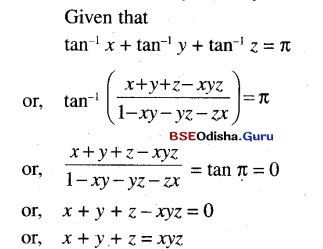(iii) tan-1 x + tan-1 y + tan-1 z = $$\frac{\pi}{2}$$. Show that xy + yz + zx = 1
Solution: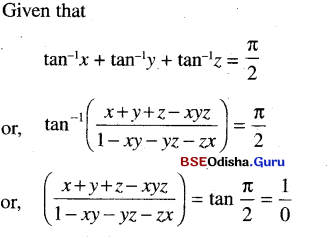or, 1 – xy – yz – zx = 0
⇒ xy + yz + zx = 1

(iv) If r2 = x2 +y2 + z2, Prove that tan-1 $$\frac{y z}{x r}$$ + tan-1 $$\frac{z x}{y r}$$ + tan-1 $$\frac{x y}{z r}$$ = $$\frac{\pi}{2}$$
Solution: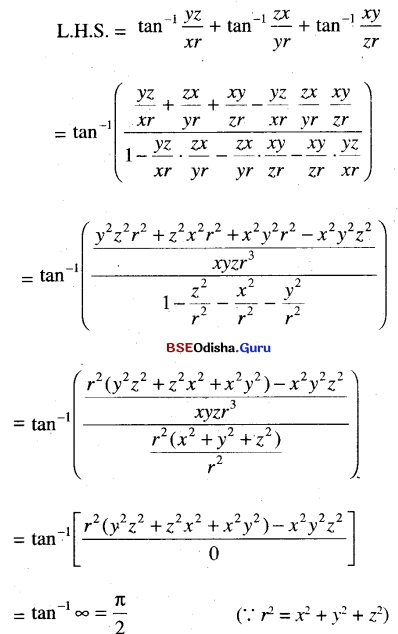(v) In a triangle ABC if m∠A = 90°, prove that tan-1 $$\frac{b}{a+c}$$ + tan-1 $$\frac{c}{a+b}$$ = $$\frac{\pi}{4}$$. where a, b, and c are sides of the triangle.
Solution:
L.H.S. tan-1 $$\frac{b}{a+c}$$ + tan-1 $$\frac{c}{a+b}$$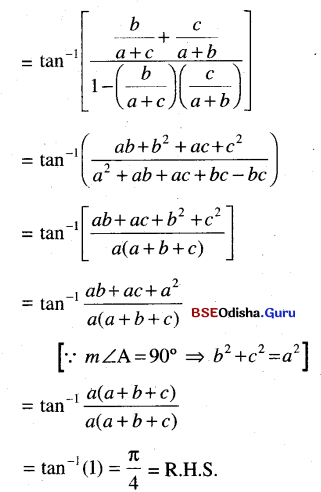Question 9.
Solve
(i) cos (2 sin-1 x) = 1/9
Solution: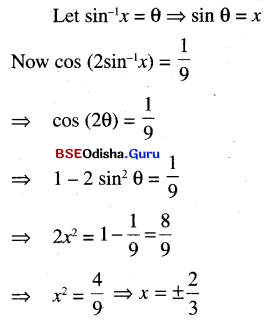(ii) sin-1 x + sin-1 (1 – x) = $$\frac{\pi}{2}$$
Solution:
sin-1 x + sin-1 (1 – x) = $$\frac{\pi}{2}$$
or, sin-1 (1 – x) = $$\frac{\pi}{2}$$ – sin-1 x = cos-1 x
or, sin-1 (1 – x) = sin-1 $$\sqrt{1-x^2}$$
or, 1 – x = $$\sqrt{1-x^2}$$
or, 1 + x2 – 2x = 1 – x2
or, 2x2 – 2x  = 0
or, 2x (x – 1) = 0
∴ x = 0 or, 1

(iii) sin-1 (1 – x) – 2 sin-1 x = $$\frac{\pi}{2}$$
Solution:
sin-1 (1 – x) – 2 sin-1 x = $$\frac{\pi}{2}$$
⇒ – 2 sin-1 x = $$\frac{\pi}{2}$$ – sin-1 (1 – x)
⇒ cos-1 (1 – x)
⇒ cos (– 2 sin-1 x) = 1 – x      ….. (1)
Let sin-1 Θ ⇒ sin Θ
Now cos (– 2 sin-1 x) = cos (-2Θ)
= cos 2Θ = 1 – 2 sin2 Θ = 1 – 2x2
Using in (1) we get
1 – 2x2 = 1 – x
⇒ 2x2 – x = 0 ⇒ x (2x – 1) = 0
⇒ x = 0, ½, But x = ½ does not
Satisfy the given equation, Thus x = 0.(iv) cos-1 x + sin-1 $$\frac{x}{2}$$ = $$\frac{\pi}{6}$$
Solution: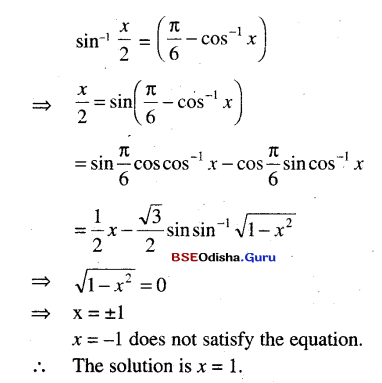(v) tan-1 $$\frac{x-1}{x-2}$$ + tan-1 $$\frac{x+1}{x+2}$$ = $$\frac{\pi}{4}$$
Solution: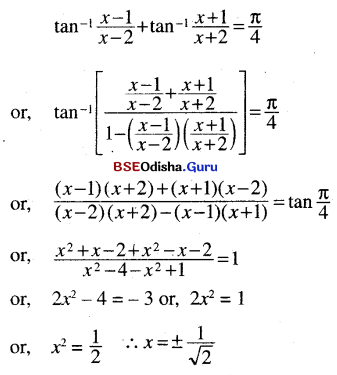(vi) tan-1 $$\frac{1}{2 x+1}$$ + tan-1 $$\frac{1}{4 x+1}$$ = tan-1 $$\frac{2}{x^2}$$
Solution: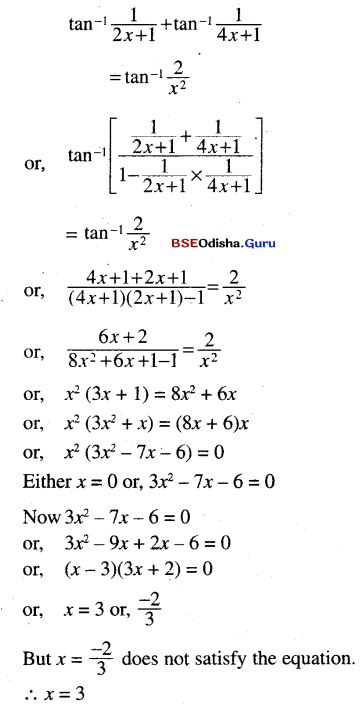(vii) 3 sin-1 $$\frac{2 x}{1+x^2}$$ – 4 cos-1 $$\frac{1-x^2}{1+x^2}$$ + 2 tan-1 $$\frac{2 x}{1-x^2}$$ = $$\frac{\pi}{3}$$
Solution: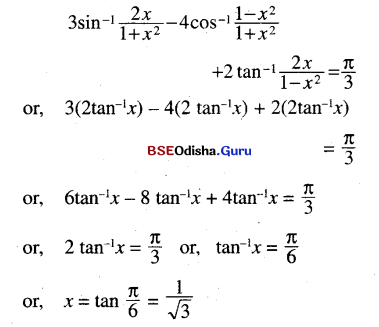(viii) cot-1 $$\frac{1}{x-1}$$ + cot-1 $$\frac{1}{x}$$ + cot-1 $$\frac{1}{x+1}$$ = cot-1 $$\frac{1}{3x}$$
Solution: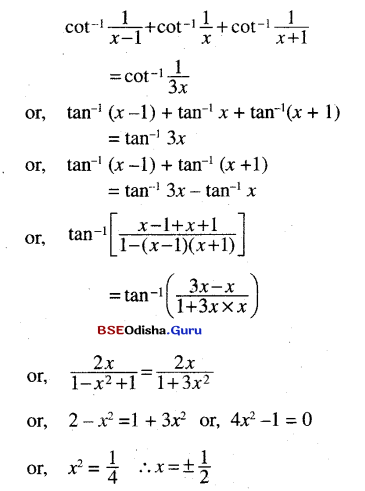(ix) cot-1 $$\frac{1-x^2}{2 x}$$ =  cosec-1 $$\frac{1+a^2}{2 a}$$ – sec-1 $$\frac{1+b^2}{1-b^2}$$
Solution: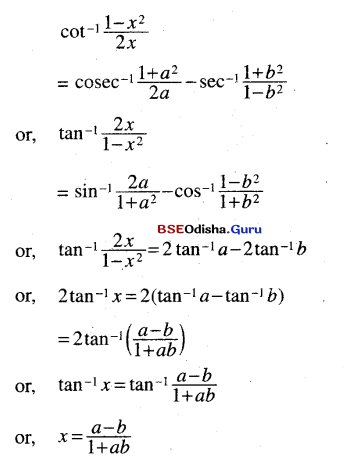(x) sin-1 $$\left(\frac{2 a}{1+a^2}\right)$$ + sin-1 $$\left(\frac{2 b}{1+b^2}\right)$$ = 2 tan-1 x
Solution: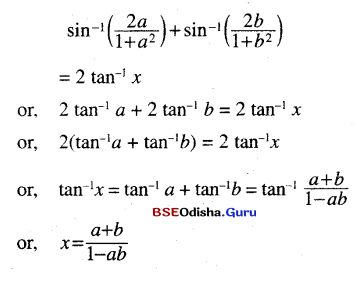(xi) sin-1 y – cos-1 x = cos-1 $$\frac{\sqrt{3}}{2}$$
Solution: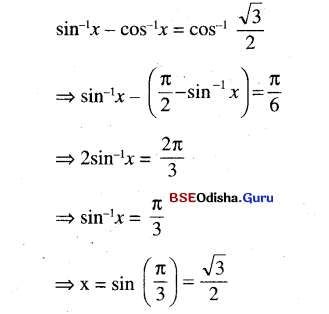(xii) sin-1 2x + sin-1 x = $$\frac{\pi}{3}$$
Solution: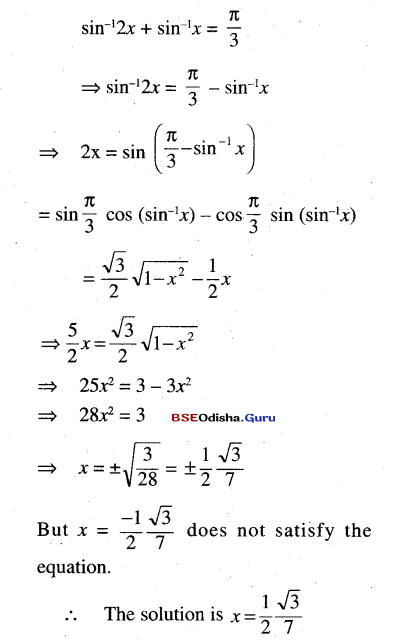Question 10.
Rectify the error ifany in the following:
sin-1 $$\frac{4}{5}$$ + sin-1 $$\frac{12}{13}$$ + sin-1 $$\frac{33}{65}$$
Solution: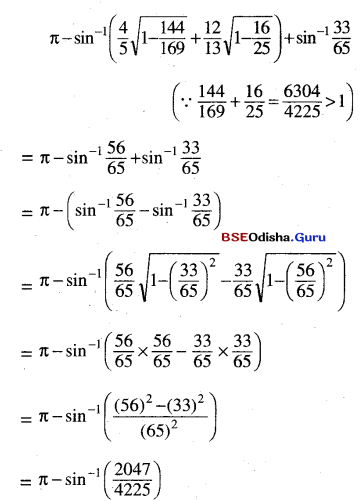Question 11.
Prove that:
(i) cos-1 $$\left(\frac{b+a \cos x}{a+b \cos x}\right)$$ = 2 tan-1 $$\left(\sqrt{\frac{a-b}{a+b}} \tan \frac{x}{2}\right)$$
Solution: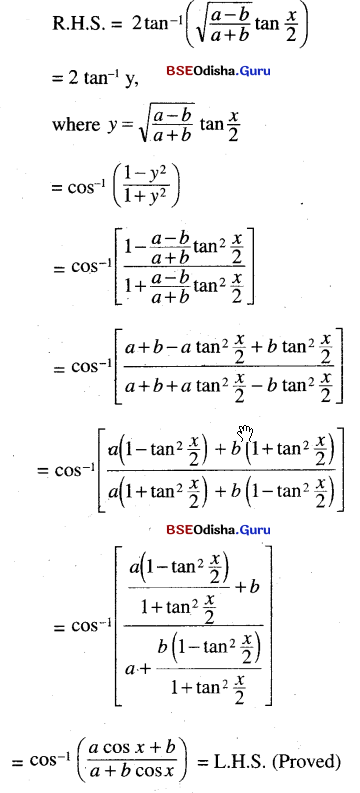(ii) tan $$\left(\frac{\pi}{4}+\frac{1}{2} \cos ^{-1} \frac{a}{b}\right)$$ + tan $$\left(\frac{\pi}{4}-\frac{1}{2} \cos ^{-1} \frac{a}{b}\right)$$
Solution: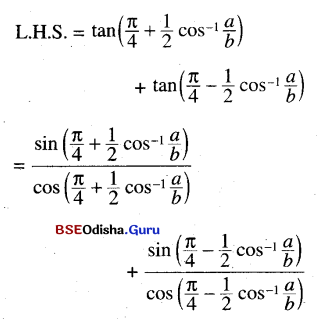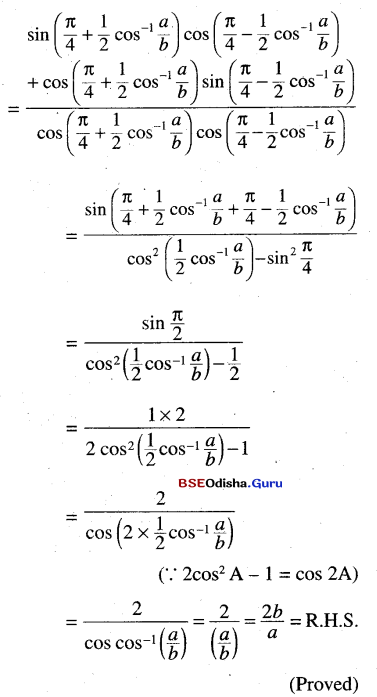(iii) tan-1 $$\sqrt{\frac{x r}{y z}}$$ + tan-1 $$\sqrt{\frac{y r}{y x}}$$ + tan-1 $$\sqrt{\frac{z r}{x y}}$$ = π where r = x + y +z.
Solution: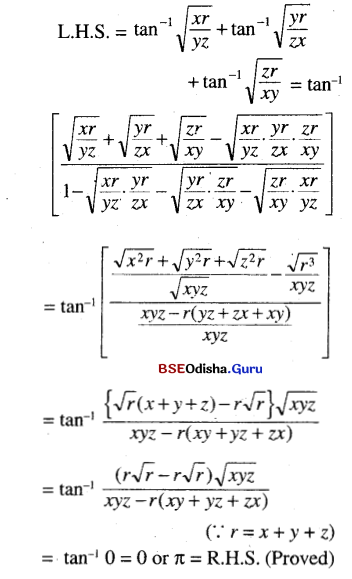Question 12.
(i) If cos-1 ($$\frac{x}{a}$$) + cos-1 ($$\frac{y}{b}$$) = Θ, prove that $$\frac{x^2}{a^2}$$ – $$\frac{2 x}{a b}$$ cos Θ + $$\frac{y^2}{b^2}$$ = sin2 Θ.
Solution: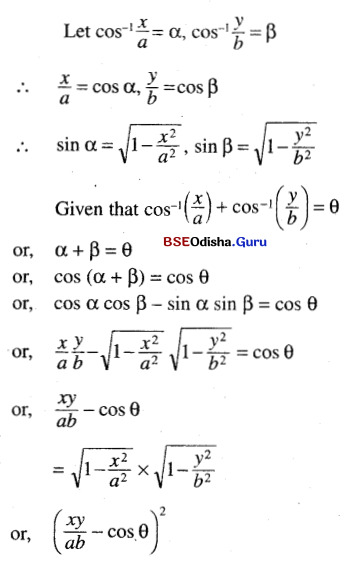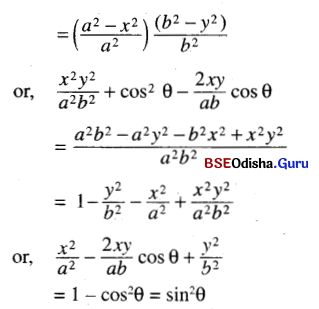(ii) If cos-1 ($$\frac{x}{y}$$) + cos-1 ($$\frac{y}{3}$$) = Θ, prove that 9x2 – 12xy cos Θ + 4y2 = 36 sin2 Θ.
Solution: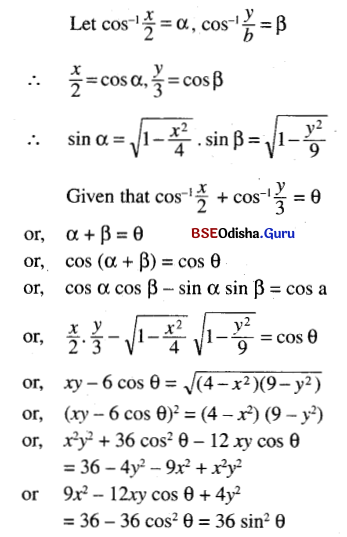(iii) If sin-1 ($$\frac{x}{a}$$) + sin-1 ($$\frac{y}{b}$$) = sin-1 ($$\frac{c^2}{a b}$$) prove that b2x2 + 2xy $$\sqrt{a^2 b^2-c^4}$$ a2y2 = c2
Solution: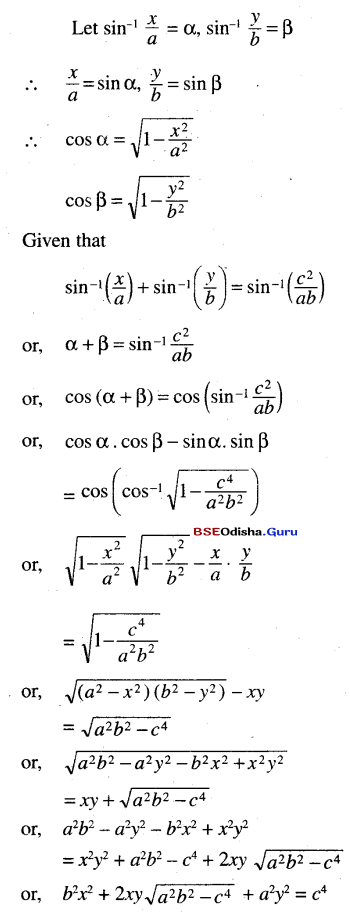(iv) If sin-1 ($$\frac{x}{a}$$) + sin-1 ($$\frac{y}{b}$$) = α prove that $$\frac{x^2}{a^2}$$ + $$\frac{2 x y}{a b}$$ cos α + $$\frac{y^2}{b^2}$$ = sin2 α
Solution: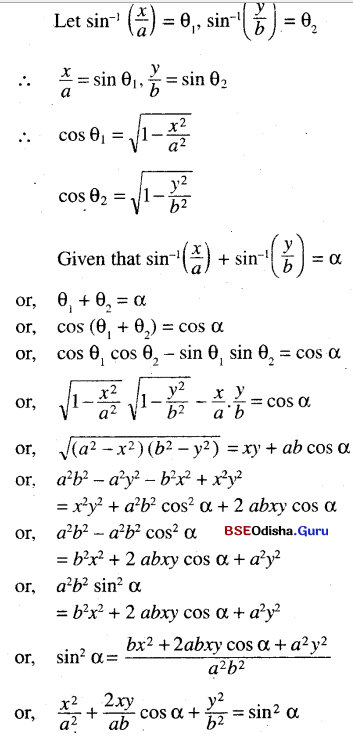(v) If sin-1 x + sin-1 y + sin-1 z = π prove that x2 + y2 + z2 + 4x2y2z2 = 2 ( x2y2 + y2z2 + z2x2 )
Solution: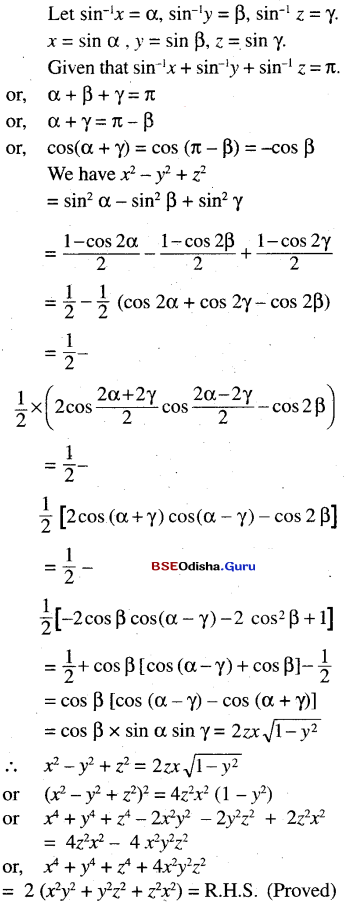Question 13.
Solve the following equations:
(i) tan-1 $$\frac{x-1}{x+1}$$ + tan-1 $$\frac{2 x-1}{2 x+1}$$ = tan-1 $$\frac{23}{36}$$
Solution: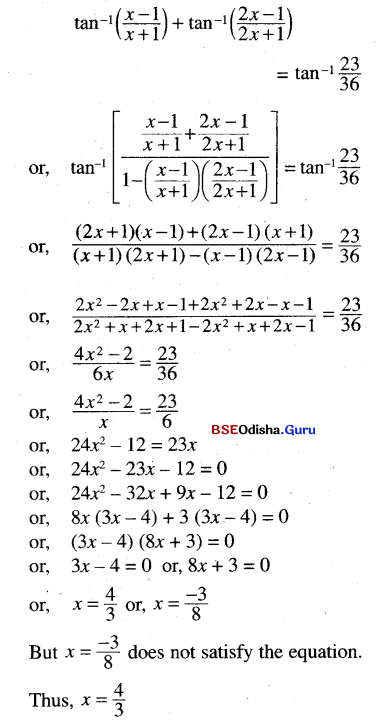(ii) tan-1 $$\frac{1}{3}$$ + tan-1 $$\frac{1}{5}$$ + tan-1 $$\frac{1}{7}$$ + tan-1 x = $$\frac{\pi}{4}$$
Solution: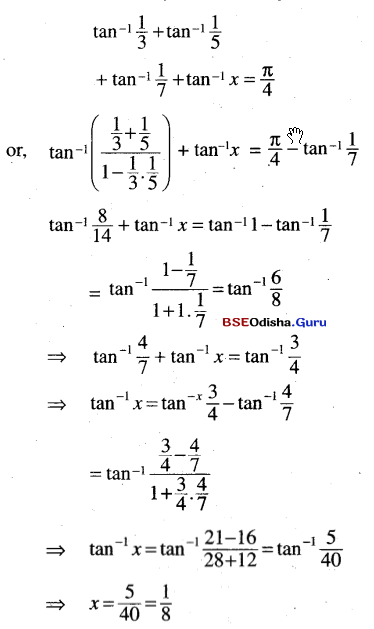(iii) cos-1 $$\left(x+\frac{1}{2}\right)$$ + cos-1 x+ cos-1 $$\left(x-\frac{1}{2}\right)$$ = $$\frac{3 \pi}{2}$$
Solution: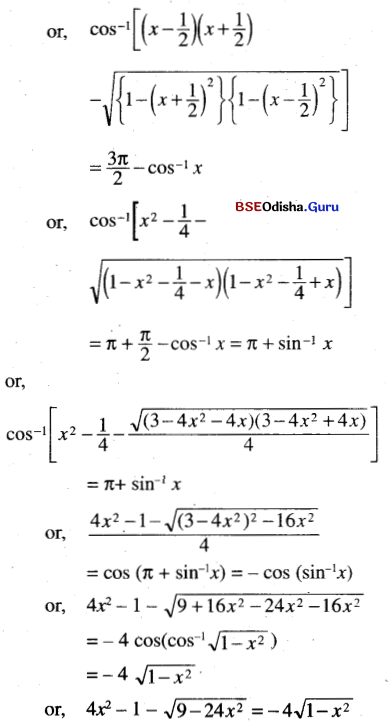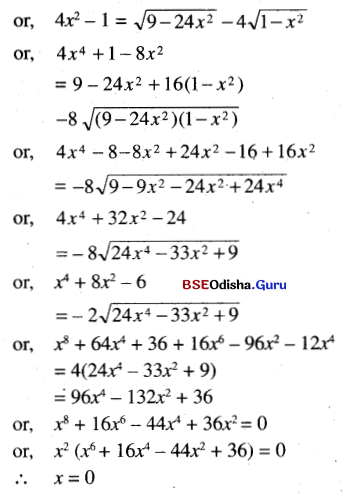(iv) 3tan-1 $$\frac{1}{2+\sqrt{3}}$$ – tan-1 $$\frac{1}{x}$$ = tan-1 $$\frac{1}{3}$$
Solution: